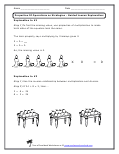Home > Topics >

## Basic Math Operations WorksheetsThese cover all the skills that overlap in the math standards. There are plenty of them too. We will be adding tons of sections that focus solely on addition, subtraction, division, and multiplication. For now a number of skills are just mashed together here. Make sure to check our word problems worksheets section for a number of problems that have similar skills.

Add and Subtraction to 5

Adding and Subtracting Within 20

Addition and Subtraction (Within 20)

Adding and Subtracting (Within 1,000)

Add and Subtract within 1000

Add and Subtract Multi-Digit Whole Numbers

Arithmetic Patterns and Systems

Basic Addition and Subtraction Word Problems

Finding Unknowns In Multiplication and Division

Fluently Adding and Subtracting

Making The Number Ten

Math Fact Families

Math Rules and Patterns

Mental Addition and Subtraction

Multiplication and Division within 100

Properties of Basic Math Operations as Strategies

Properties of Operations as Strategies

Relating Addition and Subtraction (Fact Families)

Relate Counting to Addition and Subtraction

Unknown Numbers in Sums and Differences

Word Problems That Use All Operations

Working With Equals Signs